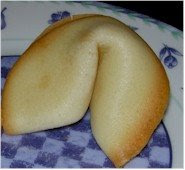## Monday, April 27, 2009

Math Circle members Dana McLaughlin and Bea Malsky came up with a very cool idea for refreshments at a recent math circle meeting: homemade fortune cookies!They used this recipe and tucked in slips of papers on which they had written various cool math puzzles, formulas and "factaroonies" before folding up the fortune cookies. It sounds like it took a bit of trial and error to get the folding technique right, but the ones they brought in looked and tasted great. (Important tip: I understand that it's easier to work the dough-folding if you make them larger than the commercial variety.)

A good place to look for cool math factaroonies to put into cookies is Tanya Khovanova's "Number Gossip" website. Type in a number, say, 28 or 17 or 13 or 27, into the Number Gossip website and you'll get a bunch of interesting facts and properties of that number. For example, lots of people know that 28 is a perfect number, but did you know that 28 is the only even perfect number of the form x3 + 1? Or that 17 is the number of different wallpaper groups (i.e., plane symmmetry groups)? Or that three planes can cut a donut into a maximum of 13 pieces? Or that someone who is 10,000 days old is age 27?

Here are a few ideas for cool formulas suitable for math fortune cookies to get you started. I'm sure you can come up with many more with a little searching:

The Euler identity: e + 1 = 0.

Euler's polyhedral formula V + E - F = 2
(where V is the number of vertices, E is the number of edges, and F is the number of faces of any given polyhedron.)

1 = 1/2 + 1/4 + 1/8 + 1/16 + 1/32 + ....

(Or equivalently, if we use "bimal" notation instead of decimal, we can write the previous equation as 1 = .1111... in bimal--the binary equivalent of decimal.)

The golden ratio phi has many beautiful equations suitable for use in a fortune cookie. Here's of them:

phi = sqrt(1 + sqrt(1 + sqrt(1 + sqrt(1 + .....

There's a wonderful world of such formulas out there! And of course, you could take the approach of posing some of these beautiful formulas in an "exploratory question" form instead of as an equation statement form.